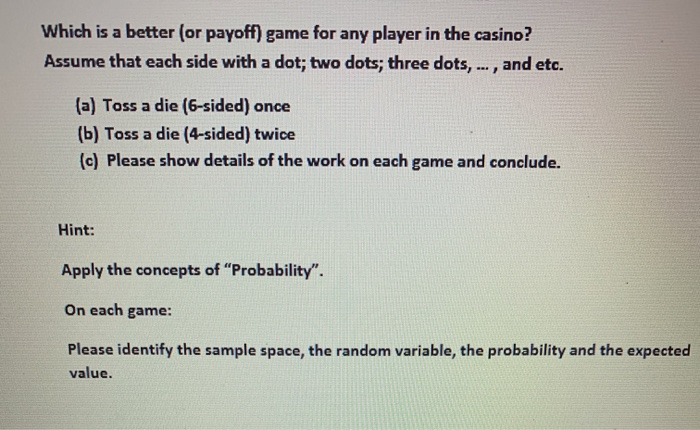# Which is a better (or payoff) game for any player in the casino? Assume that each side with a dot; two dots; three dots,., and etc. (a) Toss a die (6-sided) once (b) Toss a die (4-sided) twice (c) Pl...

Which is a better (or payoff) game for any player in the casino? Assume that each side with a dot; two dots; three dots,., and etc. (a) Toss a die (6-sided) once (b) Toss a die (4-sided) twice (c) Please show details of the work on each game and conclude.Which is a better (or payoff) game for any player in the casino? Assume that each side with a dot; two dots; three dots,... and etc. (a) Toss a die (6-sided) once (b) Toss a die (4-sided) twice (c) Please show details of the work on each game and conclude. Hint: Apply the concepts of "Probability". On each game Please identify the sample space, the random variable, the probability and the expected value.

(a) Given that a 6-sided die is tossed once.

Then the sample space S contains all six numbers, that is {1,2,3,4,5,6}

Suppose the random variable X as the pay off which is the face value that is the number obtained when the die is tossed one time.

Then values of X = 1, 2, 3, 4, 5, 6

The probability of each value of X are

P(x=1)= 1/6, P(x=2)= 1/6, P(x=3)= 1/6, P(x=4)= 1/6, P(x=5)= 1/6, P(x=6)= 1/6

Then the Expected Value is the Expected Pay Off, which is E(X) = Sum of the products of the values of X with their corresponding probabilities

= 1xP(x=1)+2xP(x=2)+3xP(x=3)+4xP(x=4)+5xP(x=5)+6xP(x=6)

=1x1/6+2x1/6+3x1/6+4x1/6+5x1/6+6x1/6

=(1+2+3+4+5+6)x1/6 = 21x1/6 = 3.5

Hence, the Expected Value is 3.5

(b)

Given that a 4-faced die is tossed twice.

Then the sample space S contains all sixteen pairs of numbers, that is {(1,1),(1,2),(1,3),(1,4),(2,1),(2,2),(2,3),(2,4), (3,1),(3,2),(3,3),(3,4), (4,1),(4,2),(4,3),(4,4)}

Suppose the random variable X as the pay off which is the sum of two face values that is the total of numbers obtained when the die is tossed twice.

Then values of X = 2, 3, 4, 5, 6, 7, 8

where

X=2 has the frequecy 1 with the pair (1,1)

X=3 has the frequecy 2 with the pairs (1,2), (2.1)

X=4 has the frequecy 3 with the pairs (1,3),( 3,1), (2,2)

X=5 has the frequecy 4 with the pairs (1,4) (4,1),(2,3), (3,2)

X=6 has the frequecy 3 with the pairs (2,4), (4,2), (3,3)

X=7 has the frequecy 2 with the pairs (3,4), (4,3)

X=8 has the frequecy 1 with the pair (4,4)

The probability of each value of X are

P(x=2)= 1/16, P(x=3)= 2/16, P(x=4)= 3/16, P(x=5)= 4/16, P(x=6)= 3/16, P(x=7)= 2/16, P(x=8)= 1/16

Then the Expected Value is the Expected Pay Off, which is E(X) = Sum of the products of the values of X with their corresponding probabilities

= 2xP(x=2)+3xP(x=3)+4xP(x=4)+5xP(x=5)+6xP(x=6)+7xP(x=7)+8xP(x=8)

=2x1/16+3x2/16+4x3/16+5x4/16+6x3/16+7x2/16+8x1/16

=(2+6+12+20+18+14+8)x1/16 = 80x1/16 = 5

Hence, the Expected Value is 5

(c) From the above two games mentioned in (a) and (b), it is obseved that the expected pay off in the game (a) is 3.5 thd the expected pay off for the game (b) is 5 therefore, the game (b) that is tossing 4-face die is better as it gives more pay off of 5 than game (a) that is tossing a 6-face die which gives less pay off of 3.5.

##### Add Answer of: Which is a better (or payoff) game for any player in the casino? Assume that each side with a dot; two dots; three dots,., and etc. (a) Toss a die (6-sided) once (b) Toss a die (4-sided) twice (c) Pl...
Similar Homework Help Questions
• ### Which is a better (or payoff) game for any player in the casino? Assume that each...Which is a better (or payoff) game for any player in the casino? Assume that each side with a dot; two dots; three dots,., and etc. (a) Toss a die (6-sided) once (b) Toss a die (4-sided) twice (c) Please show details of the work on each game and conclude. Which is a better (or payoff) game for any player in the casino? Assume that each side with a dot; two dots; three dots,... and etc. (a) Toss a die...

Free Homework App